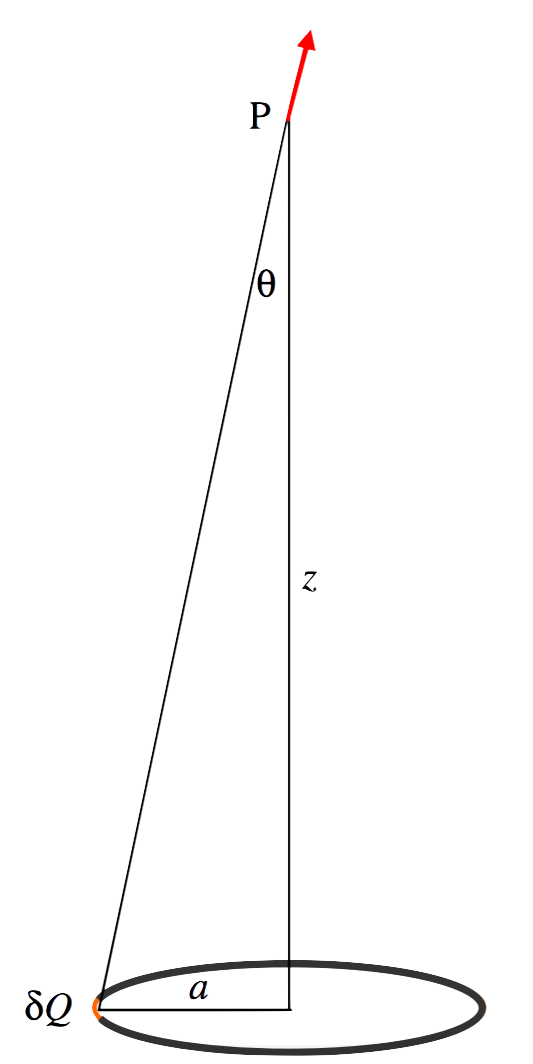$$\require{cancel}$$

# 1.6D: Field on the Axis of and in the Plane of a Charged Ring

•• Contributed by Jeremy Tatum
• Emeritus Professor (Physics & Astronomy) at University of Victoria

Field on the axis of a charged ring.Ring, radius $$a$$, charge $$Q$$. Field at P from element of charge $$δQ = \dfrac{\delta Q}{4\pi\epsilon_0 (a^2+z^2)}$$. Vertical component of this $$= \dfrac{\delta Q \cos \theta}{4\pi\epsilon_0 (a^2+z^2)}=\dfrac{\delta Qz}{4\pi\epsilon_0 (a^2+z^2)^{3/2}}$$. Integrate for entire ring:

Field $$E = \dfrac{Q}{4\pi\epsilon_0}\dfrac{z}{(a^2+z^2)^{3/2}}$$.

In terms of dimensionless variables:

$E=\dfrac{z}{(1+z^2)^{3/2}}.$

where E is in units of $$\dfrac{Q}{4\pi\epsilon_0 a^2}$$, and $$z$$ is in units of $$a$$.From calculus, we find that this reaches a maximum value of $$\dfrac{2\sqrt{3}}{9}=0.3849$$ at $$z=1/\sqrt{2}=0.7071$$.

It reaches half of its maximum value where $$\dfrac{z}{(1+z^2)^{3/2}}=\dfrac{\sqrt{3}}{9}$$.

That is, $$3-72Z+9Z^2+3Z^2=0$$, where $$Z=z^2$$.

The two positive solution are $$Z = 0.041889 \text{ and }3.596267$$.

That is, $$z = 0.2047 \text{ and }1.8964$$.

Field in the plane of a charged ring.

We suppose that we have a ring of radius $$a$$ bearing a charge $$Q$$. We shall try to find the field at a point in the plane of the ring and at a distance $$r (0 ≤ r < a)$$ from the centre of the ring.Consider an element $$δθ$$ of the ring at P. The charge on it is $$\dfrac{Q\delta \theta}{2\pi}$$. The field at A from this element of charge is

$\dfrac{1}{4\pi\epsilon_0}\cdot \dfrac{Q\delta\theta}{2\pi}\cdot \dfrac{1}{a^2+r^2-2ar\cos \theta}=\dfrac{Q}{4\pi\epsilon_0 .2\pi a^2}\cdot \dfrac{\delta\theta}{b-c\cos \theta},$

where $$b=1+r^2/a^2$$ and $$c = 2r / a$$. The component of this toward the centre is

$$-\dfrac{Q}{4\pi\epsilon_0 .2 \pi a^2}\cdot\dfrac{\cos \phi \delta\theta}{b-c\cos \theta}$$.

To find the field at A due to the entire ring, we must express $$\phi$$ in terms of $$θ$$, $$r$$ and $$a$$, and integrate with respect to $$θ \text{ from }0 \text{ to }2π$$ (or from $$0 \text{ to }π$$ and double it). The necessary relations are

$p^2=a^2+r^2-2ar\cos \theta$

$\cos \phi = \dfrac{r^2+p^2-a^2}{2rp}.$

The result of the numerical integration is shown below, in which the field is expressed in units of $$Q/(4\pi\epsilon_0 a^2)$$ and $$r$$ is in units of $$a$$.